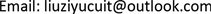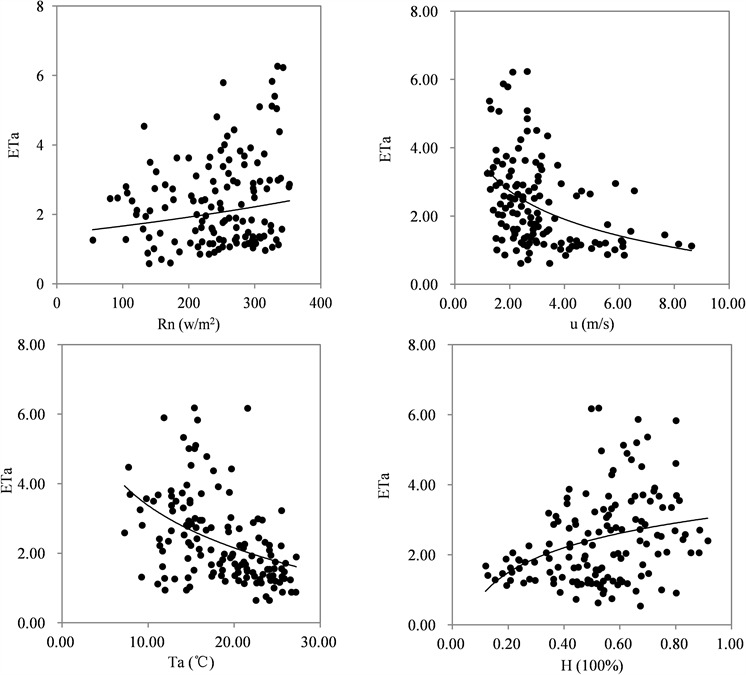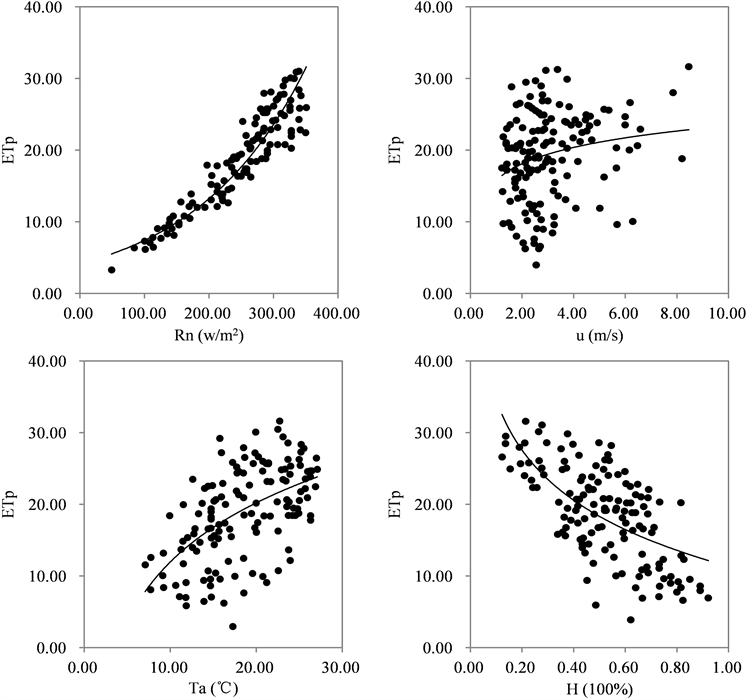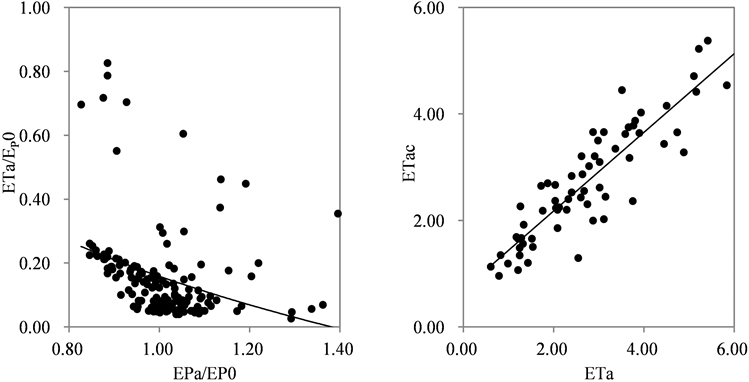﻿ 半干旱地区蒸发互补关系 Analysis of Evaporation Complementary Relationship in Semi-Arid Areas

Geographical Science Research
Vol. 08  No. 04 ( 2019 ), Article ID: 33120 , 7 pages
10.12677/GSER.2019.84038

Analysis of Evaporation Complementary Relationship in Semi-Arid Areas

Ziyu Liu

School of Atmospheric Sciences, Chengdu University of Information Technology, Chengdu SichuanReceived: Nov. 1st, 2019; accepted: Nov. 18th, 2019; published: Nov. 25th, 2019ABSTRACT

Using the net radiation, latent heat flux, average wind speed, average temperature and average relative humidity data of Yuzhong County from 2006 to 2009, the effects of meteorological factors on actual evapotranspiration, possible evapotranspiration and wet evapotranspiration were studied and the relative actual evapotranspiration was used. An estimated model of the actual evapotranspiration is established relative to the possible evapotranspiration. The study found that in the course of daily changes, the actual evapotranspiration, possible evapotranspiration, and evapotranspiration in the humid environment showed a trend of increasing first and then decreasing. There is an important influence on the actual evapotranspiration. The meteorological factor is the near-surface temperature, showing a negative correlation. The main influencing factor of the evapotranspiration in the humid environment is the meteorological factor of ground net radiation, showing a positive correlation. An estimation model of the actual evapotranspiration (A = 0.834, B = −0.664) was further established, and the model was proved to have practical availability.

Keywords:Possible Evapotranspiration, Wet Evapotranspiration, Actual Evapotranspiration Estimation Model1. 引言

2. 资料和方法

2.1. 资料

2.2. 方法

${r}_{kl}=\frac{1}{n}\underset{i=1}{\overset{n}{\sum }}\left(\frac{{x}_{ki}-{x}_{k}}{{s}_{k}}\right)\left(\frac{{x}_{li}-{x}_{l}}{{s}_{l}}\right)$ (1)

3. 结果和分析

3.1. 蒸散量日变化特征分析Figure 1. Diurnal variation of actual evapotranspiration ( ${E}_{Ta}$ ), possible evapotranspiration ( ${E}_{pa}$ ) and wet evapotranspiration ( ${E}_{p0}$ )

3.2. 实际蒸散量湿润环境蒸散量与气象因子的关系Figure 2. Correlation between actual evapotranspiration and Rn, U, T, HFigure 3. Correlation between evapotranspiration and Rn, U, T and H in humid environment

3.3. 实际蒸散量的估算模型建立

${E}_{Ta}=\left(A+B\alpha \right)\frac{\Delta }{\Delta +\gamma }\left({R}_{n}-G\right)+A\frac{\gamma }{\Delta +\gamma }{E}_{a}$ (2)

${E}_{Ta}=0.35\frac{\Delta }{\Delta +\gamma }\left({R}_{n}-G\right)-0.664\frac{\gamma }{\Delta +\gamma }{E}_{a}$ (3)Figure 4. Correlation diagram between the actual evapotranspiration and the relative evapotranspiration, the actual evapotranspiration and the estimated actual evapotranspiration

4. 结论

1) 在日变化的过程中，受地面所接受净辐射、潜热通量、空气湿度、温度等因素的影响，实际蒸散量、可能蒸散量以及湿润环境蒸散量随陆面热量的增加和温度的升高呈现出先升高、后下降的日变化特征。可能蒸散量在先上升后下降的基础上，一直大于实际蒸散量和湿润环境蒸散量。

2) 根据各气象因子与实际蒸散量和湿润环境蒸散量的相关性分析，可以得到，潜热通量是影响实际蒸散量的主要气象因子，呈现负相关关系，相关系数为0.533，地面净辐射是影响湿润环境蒸散量的主要气象因子，在置信度为0.01下正相关性显著，相关系数为0.928。

3) 利用相对实际蒸散量和相对可能蒸散量建立起实际蒸散量的估算模型

${E}_{Ta}=0.35\frac{\Delta }{\Delta +\gamma }\left({R}_{n}-G\right)-0.664\frac{\gamma }{\Delta +\gamma }{E}_{a}$，并检验所得实际蒸散量估算式精确度，得到实际蒸散量与估算蒸散量的相关系数为0.941，均方根误差RMSE = 0.434，故该模型通过检验。

Analysis of Evaporation Complementary Relationship in Semi-Arid Areas[J]. 地理科学研究, 2019, 08(04): 363-369. https://doi.org/10.12677/GSER.2019.84038

1. 1. 刘建梅, 王安志, 刁一伟, 等. 分布式模型在流域蒸散模拟中的应用与验证[J]. 应用生态学报, 2006, 17(1): 16-24.

2. 2. 冯国章. 区域蒸散发量的气候学计算方法[J]. 水文, 1994(3): 7-11+65.

3. 3. 韩松俊, 胡和平, 田富强. 三种通过常规气象变量估算实际蒸散量模型的适用性比较[J]. 水利学报, 2009, 40(1): 75-81.

4. 4. 谢平, 陈晓宏, 王兆礼, 等. 东江流域实际蒸发量与蒸发皿蒸发量的对比分析[J]. 地理学报, 2009, 64(3): 270-277.

5. 5. 李鹏飞, 孙小明, 赵昕奕. 近50年中国干旱半干旱地区降水量与潜在蒸散量分析[J]. 干旱区资源与环境, 2012, 26(7): 57-63.

6. 6. Zhang, Y., Liu, C., Tang, Y., et al. (2007) Trends in Pan Evaporation and Reference and Actual Evapotranspiration across the Tibetan Plateau. Journal of Geophysical Research, 112, D12110.
https://doi.org/10.1029/2006JD008161

7. 7. Thornthwaite, C.W. (1948) An Approach toward a Rational Classi-fication of Climate. Geographical Review, 38, 55-94.
https://doi.org/10.2307/210739

8. 8. Priestley, C.H.B. (1972) On the Assessment of Surface Heat Flux and Evaporation Using Large-Scale Parameters. Monthly Weather Review, 100, 81-92.
https://doi.org/10.1175/1520-0493(1972)100<0081:OTAOSH>2.3.CO;2

9. 9. Davies, J.A. and Allen, C.D. (2010) Equilibrium, Potential and Actual Evaporation from Cropped Surfaces in Southern Ontario. Journal of Applied Meteorology, 12, 1312-1321.

10. 10. Burutsaert, W. (1979) An Advection Aridity Approach to Estimate Actual Regional Evapotranspiration. Water Resources Research, 15, 443-450.
https://doi.org/10.1029/WR015i002p00443

11. 11. 邱新法, 曾燕, 缪启龙, 等. 用常规气象资料计算陆面年实际蒸散量[J]. 中国科学地球科学, 2003, 33(3): 281-288.

12. 12. 易诗雅. 《中国国家地理》办刊特征研究[D]: [硕士学位论文]. 长沙: 湖南师范大学, 2014.

13. 13. 徐敏, 朱永华, 李源, 等. 淮北平原实际蒸散量计算及时空分布特征分析[J]. 水电能源科学, 2016(1): 10-14.

14. 14. 刘钰, Pereira, L.S., Teixeira, J.L., 等. 参照腾发量的新定义及计算方法对比[J]. 水利学报, 1997(6): 27-33.

15. 15. 刘昌明, 张丹. 中国地表潜在蒸散发敏感性的时空变化特征分析[J]. 地理学报, 2011, 66(5): 579-588.